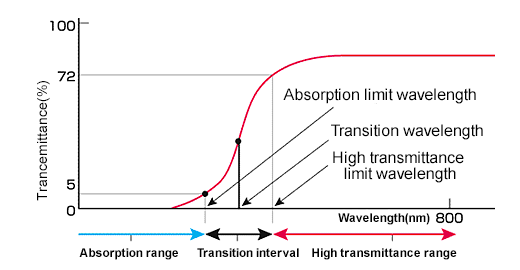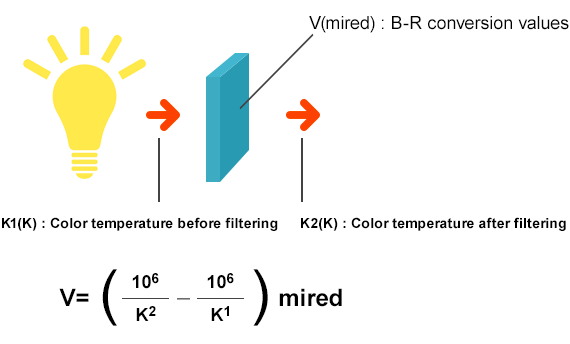Colored Glass Filters

COLORED GLASS FILTERS

Explanation of Glass Characteristics (Transmittance Glossary)

λT (nm) Transition wavelength Midpoint between wavelength with 5% of transmittance and wavelength with 72% of transmittance Transition interval Distance between wavelength with 5% of transmittance and wavelength with 72% of transmittance Transmittance in the high transmittance range Average transmittance in the range from wavelength with 72% of transmittance to wavelength of 800 nm.Wavelength with [5%] of transmittance Wavelength with [80%] of transmittance Average transmittance. Average value of transmittances in steps of 10 nm from 400 to 700 nm. Maximum transmittance. Transmittance of wavelength at maximum transmittance. Less than 1% wavelength at short wave side. Less than 5% wavelength at long wave side Transmittance at 254 nm Transmittance at 405 nm Transmittance at 435 nm Transmittance at 445 nm Transmittance at 550 nm Transmittance at 586 nm Transmittance at 750 nm Transmittance at 1000 nm B-R conversion values For color photography, the Japanese Industrial Standards JIS B 7125, ″Photographic Light-Balancing Glass Filters″, specify the values for general photographic lighting. The B-R conversion values of filters are calculated by the formula as follows: V = 221 (Log 10 Tr - Log 10 Tb) mired * Tr: The average value (%) of transmittances of the filter at 610 nm, 635 nm, and 655 nm of wavelengths.* Tb: The average value (%) of transmittances of the filter at 405 nm, 435 nm, and 465 nm of wavelengths. ■ Relationship among color temperature before filtering, color temperature after filtering and B-R conversion values* The relationship may slightly deviate from the formula described above depending on the transmittances of the light source.